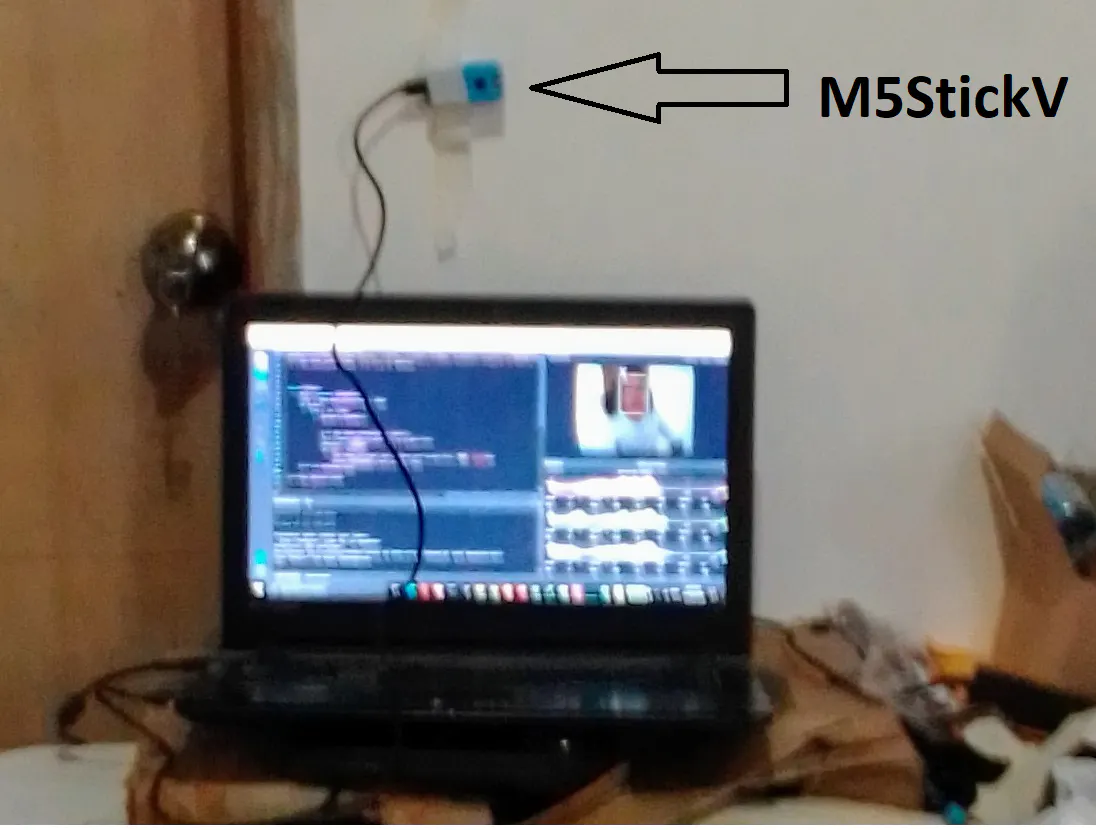# Mobile Social Distancing using Face detection (M5stickV)

Mountable device for your Personal AI Social Distancing Alarm by detecting how far the person's face from you..... (M5StickV)

IntermediateShowcase (no instructions)1 hour759## Things used in this project

### Hardware componentsM5Stack M5StickV K210 AI Camera (Without Wifi)
×1

 MaixPy

## Schematics

### PC + M5stickV## Code

### Social Distancing Alarm

Python
```# personal social distnce alarm
#average w 30 h 33 at 1.5 meters apprx...

import sensor
import image
import lcd
import KPU as kpu
import math

from fpioa_manager import *
from Maix import GPIO
from board import board_info

fm.register(board_info.LED_W, fm.fpioa.GPIO3)
led_w = GPIO(GPIO.GPIO3, GPIO.OUT)
led_w.value(1) # LED is Active Low

fm.register(board_info.LED_R, fm.fpioa.GPIO4)
led_r = GPIO(GPIO.GPIO4, GPIO.OUT)
led_r.value(1) # LED is Active Low

fm.register(board_info.LED_G, fm.fpioa.GPIO5)
led_g = GPIO(GPIO.GPIO5, GPIO.OUT)
led_g.value(1) # LED is Active Low

fm.register(board_info.LED_B, fm.fpioa.GPIO6)
led_b = GPIO(GPIO.GPIO6, GPIO.OUT)
led_b.value(1) # LED is Active Low

img = image.Image()
lcd.init()
sensor.reset()
sensor.set_pixformat(sensor.RGB565)
sensor.set_framesize(sensor.QVGA)
sensor.run(1)
from array import *

anchor = (1.889, 2.5245, 2.9465, 3.94056, 3.99987, 5.3658, 5.155437, 6.92275, 6.718375, 9.01025)
a = kpu.init_yolo2(task, 0.5, 0.3, 5, anchor)

while(True):
img = sensor.snapshot()
if code:
for i in code:
print(i.index())

a = img.draw_rectangle(i.rect())
Vio = math.sqrt((i.h()**2) + (i.w()**2))
message = "safe"
if (46 < Vio) :
img.draw_string(i.x(), int(i.y()/(i.h()/4)), "X", scale=3)
print("Not Safe",i.w(),i.h(), Vio)
led_w.value(1)
led_r.value(0)
led_g.value(1)
led_b.value(1)
led_w.value(1)
led_r.value(1)
led_g.value(1)
led_b.value(1)

a = lcd.display(img)